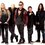# Calculus problem

$\large \dfrac d{dx} \int_{t^2}^{x^3} \dfrac 1{\sqrt{x^2+t^2}} \, dt = \, ?$Note by Ujjwal Mani Tripathi
4 years, 10 months ago

This discussion board is a place to discuss our Daily Challenges and the math and science related to those challenges. Explanations are more than just a solution — they should explain the steps and thinking strategies that you used to obtain the solution. Comments should further the discussion of math and science.

When posting on Brilliant:

• Use the emojis to react to an explanation, whether you're congratulating a job well done , or just really confused .
• Ask specific questions about the challenge or the steps in somebody's explanation. Well-posed questions can add a lot to the discussion, but posting "I don't understand!" doesn't help anyone.
• Try to contribute something new to the discussion, whether it is an extension, generalization or other idea related to the challenge.

MarkdownAppears as
*italics* or _italics_ italics
**bold** or __bold__ bold
- bulleted- list
• bulleted
• list
1. numbered2. list
1. numbered
2. list
Note: you must add a full line of space before and after lists for them to show up correctly
paragraph 1paragraph 2

paragraph 1

paragraph 2

[example link](https://brilliant.org)example link
> This is a quote
This is a quote
    # I indented these lines
# 4 spaces, and now they show
# up as a code block.

print "hello world"
# I indented these lines
# 4 spaces, and now they show
# up as a code block.

print "hello world"
MathAppears as
Remember to wrap math in $$ ... $$ or $ ... $ to ensure proper formatting.
2 \times 3 $2 \times 3$
2^{34} $2^{34}$
a_{i-1} $a_{i-1}$
\frac{2}{3} $\frac{2}{3}$
\sqrt{2} $\sqrt{2}$
\sum_{i=1}^3 $\sum_{i=1}^3$
\sin \theta $\sin \theta$
\boxed{123} $\boxed{123}$

Sort by:

- 4 years, 10 months ago

yeah but i am still not able to get the required answer will you please post the solution to this question...

- 4 years, 10 months ago

The links pretty spells out the solution already. If you really can't solve it, why don't you post your attempt here and I'll see where you are stuck on.

- 4 years, 10 months ago

i am getting the answer $\dfrac{3x^2}{\sqrt{x^2 + x^12 }}$ it is x^12 .. in the denominator

- 4 years, 10 months ago

Split the integral into $\int_0^{x^3} - \int_0^{t^2}$. For the first integral, just apply the fundamental theorem of calculus. For the second theorem, you will get some constant number, so you can ignore it after you differentiate. Is this enough information for you?

- 4 years, 10 months ago

Yeah that is enough

- 4 years, 10 months ago

- 4 years, 10 months ago

you have to develop the steps...

- 4 years, 10 months ago

do you mean that i really need to integrate the above expression first?

- 4 years, 10 months ago

It's not necessary, but if you make the change $t = x \cdot \text{ Sh u}$ with $x$ constant, and use $1 + Sh^2(u) = Ch^2(u)$ and $dt = x \cdot \text{Ch u} \space du$, the problemm looks like easy. Tomorrow, I promise you'll have my answer, now I'm very busy, Im' leaving my house. Tomorrow, I promise....

- 4 years, 10 months ago

thanks man , appreciate the help

- 4 years, 10 months ago

Ok, come on, please tell me if I'm wrong, I'm a person and due to this I make a lot of(too many) mistakes...

Proof 1 (attempt 1).-

$\dfrac d{dx} \int_{t^2}^{x^3} \dfrac 1{\sqrt{x^2 + t^2}} \, dt = \dfrac d{dx} \left( \int_{0}^{x^3} \dfrac 1{\sqrt{x^2 + t^2}} \, dt - \int_{0}^{t^2} \dfrac 1{\sqrt{x^2 + t^2}} \, dt \right) =$ Let's call $g(x) = x^3$ and $f(x) = \int_{0}^{x} \dfrac 1{\sqrt{x^2 + t^2}} \, dt$ Then, $\dfrac d{dx} \int_{0}^{x^3} \dfrac 1{\sqrt{x^2 + t^2}} \, dt = \dfrac d{dx} f(g(x)) = \dfrac d{dx} f(x^3) = 3x^2 \cdot ...$ Sorry, I'm getting a knot for myself...

Proof 2.-

$\dfrac d{dx} \int_{t^2}^{x^3} \dfrac 1{\sqrt{x^2 + t^2}} \, dt = \space (*)$ Let's make the change $t = x \cdot \text{ Sh u}$ , $x$ constant, $dt = x \cdot \text{Ch u} \space du$ $(*) = \dfrac d{dx} \int_{Sh^{-1}(x \cdot \text{ Sh}^{2}u)}^{Sh^{-1} x^2} \, \pm \space du =...$ To be continued

- 4 years, 10 months ago

Hmm it's getting better

- 4 years, 10 months ago

I think the rest proof 2 should be simple, I'll finish it. I promise.... but you have to say me wheteher I'm right or not... It depends also if $x >0$, $x = 0$ or $x < 0$ to concrete the ending. This question is giving me questions and suggestions... For example, does $\int_0^{t} \space dt$ make some sense?If the answer is yes, Is it $t$?why?... I have another proof making the change $u = \sqrt{x^2 + t^2} + t$... Even we can think first this question without the derivative $\frac{d}{dx}$, I mean given $x$ possible concrete constants, $x =1$, $x =2$, $x = 100$... and later, seeing the results, infering the last answer... And I'm also considering Pi's advice: Use fundamental theorem of Calculus... To be continued... Can you finish my 2nd proof, and I tell you what I think about your result? Come on, dare...

- 4 years, 10 months ago

Make the change I have said to you... It must solve it quickly.. or use Fundamental theorem of calculus

- 4 years, 10 months ago

can you provide the final answer , i am really stuck on this ?

- 4 years, 10 months ago

@Pi Han Goh @Guillermo Templado help will be welcomed .

- 4 years, 10 months ago

I agree with Pi Hang Goh, you can also make the change $t = x\Sh(u)$ solve , the integral, and later derivate

- 4 years, 10 months ago

I mean $t = x \cdot \text{Sh u}$. I have some problems with my comments..

- 4 years, 10 months ago

Tomorrow, I rewiewed this, and if it's not solved. I'll try to solve it, I think I'm not going to have a lot problemms.. we'll see...

- 4 years, 10 months ago